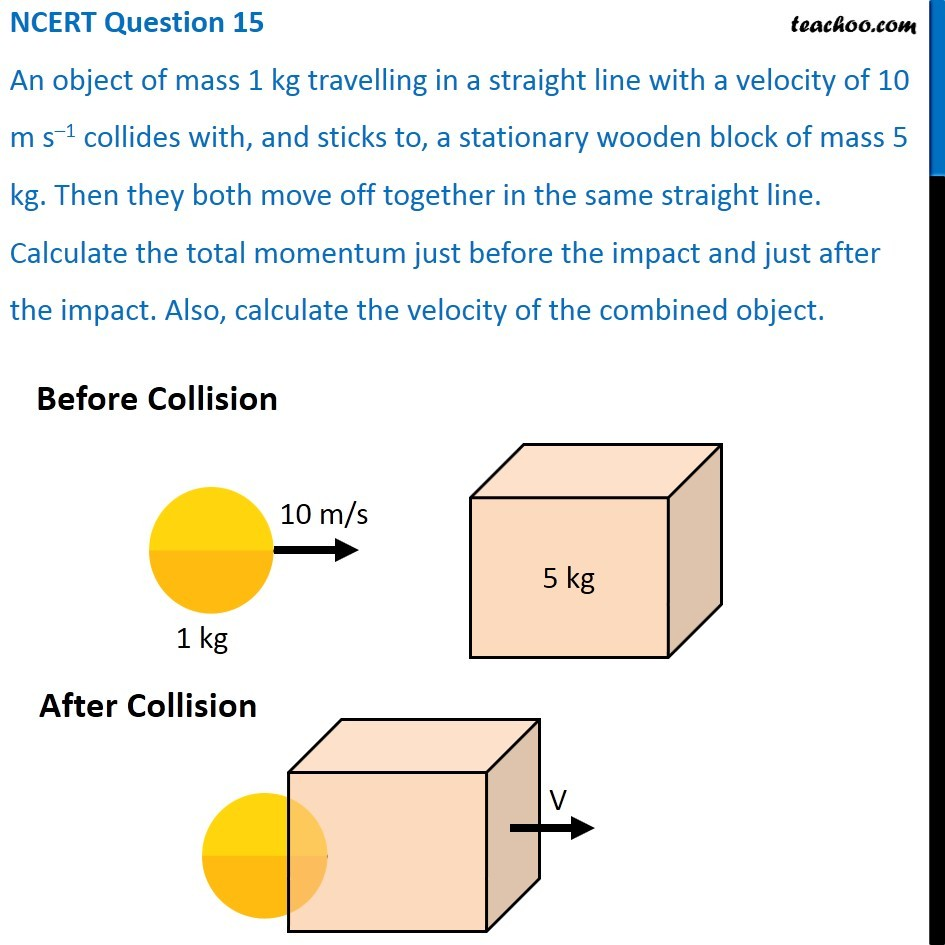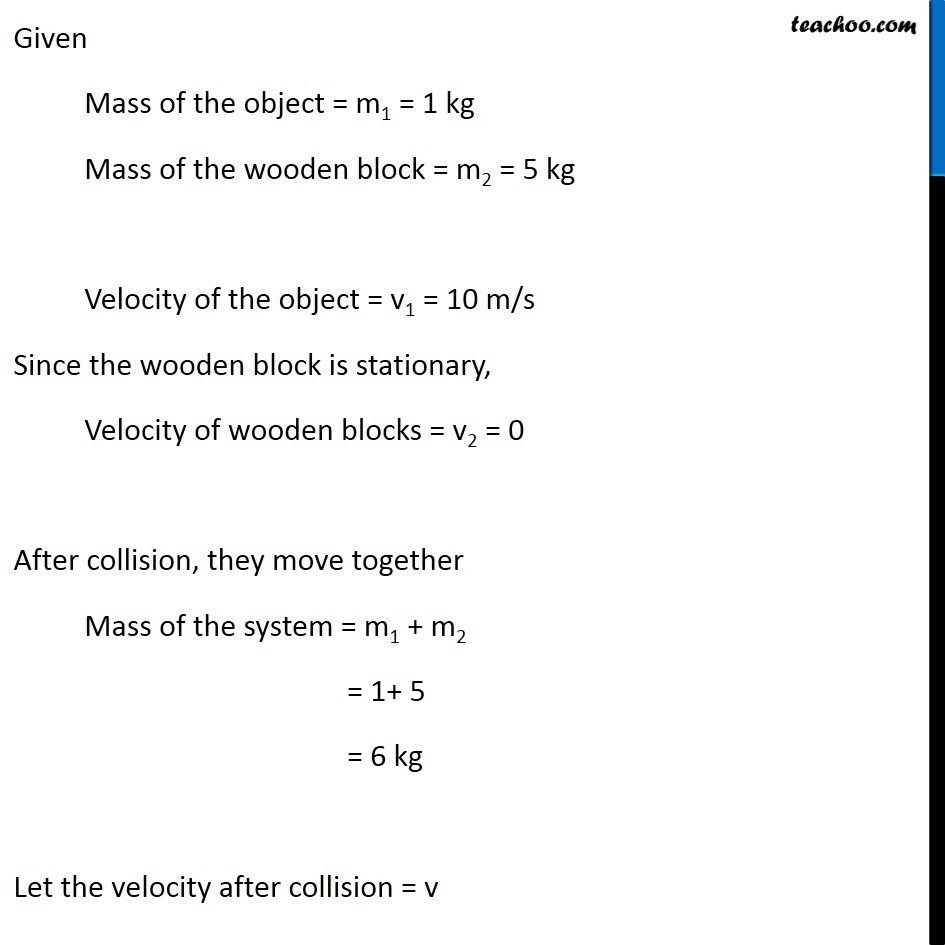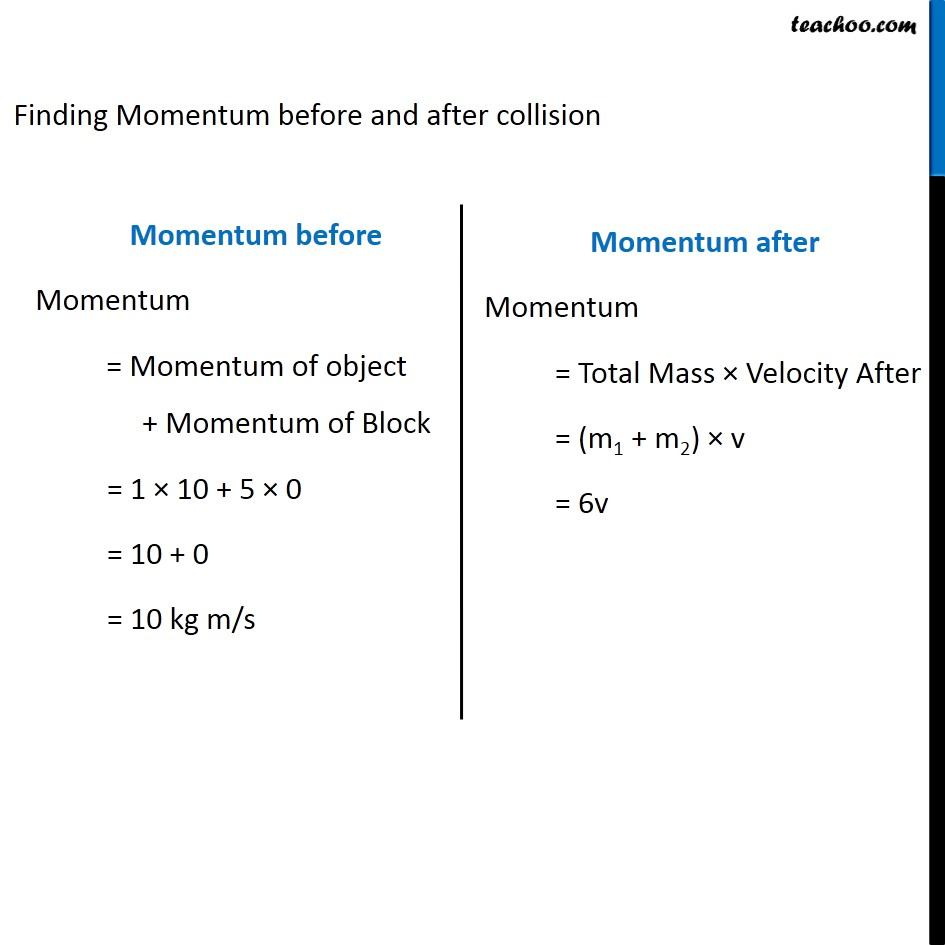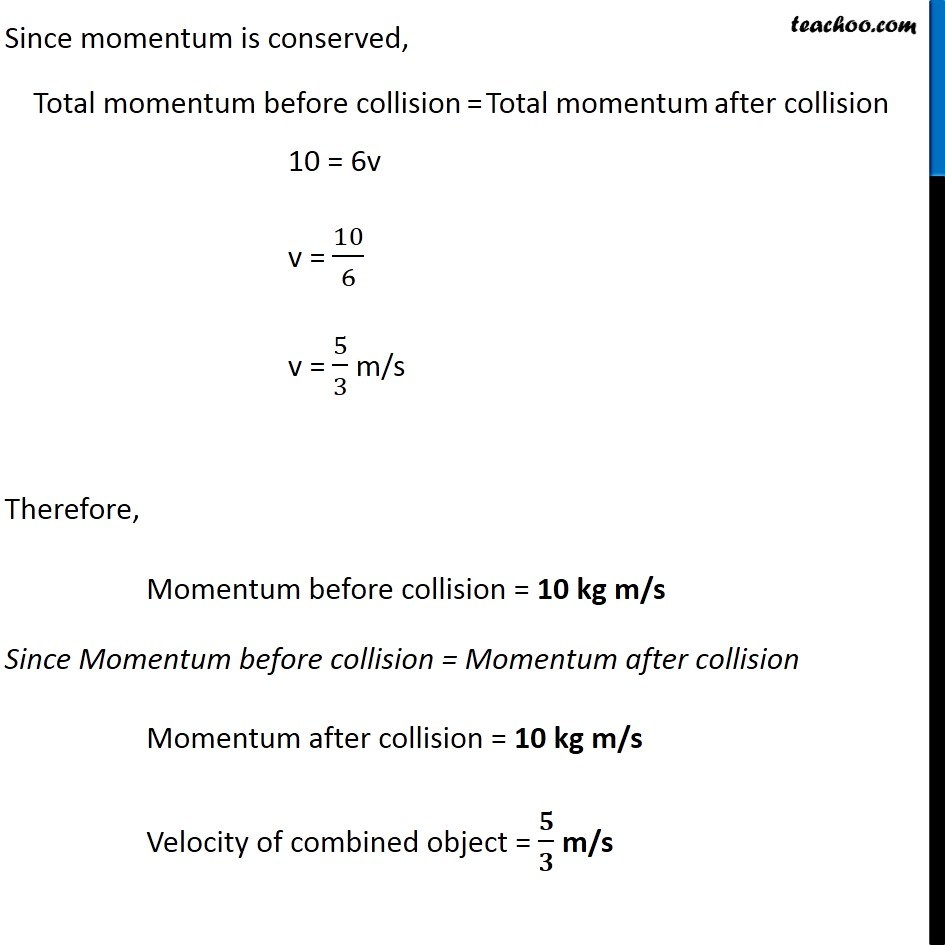NCERT Questions

Class 9
Chapter 9 Class 9 - Force and Laws Of MotionGet live Maths 1-on-1 Classs - Class 6 to 12

### Transcript

NCERT Question 15 An object of mass 1 kg travelling in a straight line with a velocity of 10 m s–1 collides with, and sticks to, a stationary wooden block of mass 5 kg. Then they both move off together in the same straight line. Calculate the total momentum just before the impact and just after the impact. Also, calculate the velocity of the combined object. Before Collision After Collision Given Mass of the object = m1 = 1 kg Mass of the wooden block = m2 = 5 kg Velocity of the object = v1 = 10 m/s Since the wooden block is stationary, Velocity of wooden blocks = v2 = 0 After collision, they move together Mass of the system = m1 + m2 = 1+ 5 = 6 kg Let the velocity after collision = v Finding Momentum before and after collision Momentum before Momentum = Momentum of object + Momentum of Block = 1 × 10 + 5 × 0 = 10 + 0 = 10 kg m/s Momentum after Momentum = Total Mass × Velocity After = (m1 + m2) × v = 6v Since momentum is conserved, Total momentum before collision = Total momentum after collision 10 = 6v v = 10/6 v = 5/3 m/s Therefore, Momentum before collision = 10 kg m/s Since Momentum before collision = Momentum after collision Momentum after collision = 10 kg m/s Velocity of combined object = 𝟓/𝟑 m/s